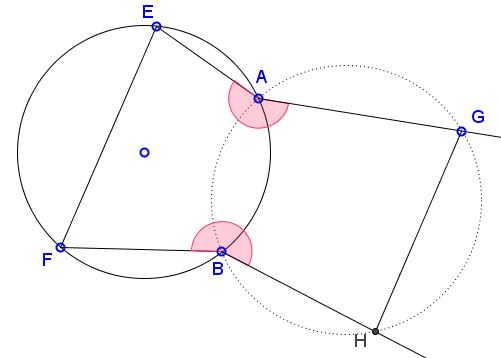# Reim's Similar Coins IV

### Problem

Given a cyclic quadrilateral $ABFE,$ let $AG$ and $BH$ be extended away from $ABFE$ so that

1. $\angle EAG=\angle FBH,$ but measured in opposite directions,
2. $GH\parallel EF.$Then quadrilateral $ABHG$ is cyclic.

### Solution

Draw circle $(ABG).$ We need to show that $H$ lies on that circle.

Find $G'$ on $(ABG)$ such that $AG'\parallel FB.$ Add $H'$ on $(ABG),$ with $G'H'\parallel EF.$ By one of Reim's theorems, $FH'$ passes through $B.$ By the construction, $\angle FBH'=\angle EAG'$ so that also $\angle HBH'=\angle GAG'.$If $H''$ is the intersection of $GH$ with circle $(ABG)$ then $\overparen{H'H''}=\overparen{GG'}$ from which $GH''\parallel G'H',$ implying $H=H''.$ It follows that the points $A,B,H,G$ are indeed concyclic.

### Chasing Inscribed Angles